# Garret Cahill

•### Graphing Quadratic Equations - Homework 1

Activity

Garret Cahill

•### Systems of Inequalities - HW1

Activity

Garret Cahill

•### Graphing Quadratic Equations - Classwork Example 4

Activity

Garret Cahill

•### Graphing Quadratic Equations - Classwork Example 3

Activity

Garret Cahill

•### Graphing Quadratic Equations - Classwork Example 2

Activity

Garret Cahill

•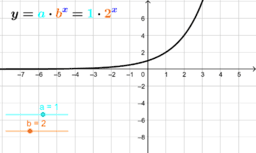### Exploring Graphs of Exponential Functions

Activity

Garret Cahill

•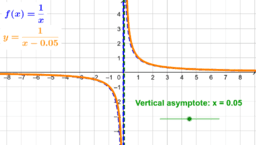### Visualizing Vertical Asymptote

Activity

Garret Cahill

•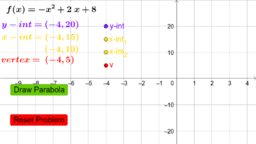### Graphing Quadratic Equations - Exam

Activity

Garret Cahill

•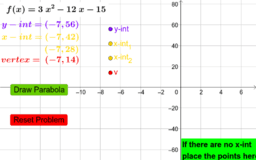### Graphing Quadratic Equations - Practice Exam

Activity

Garret Cahill

•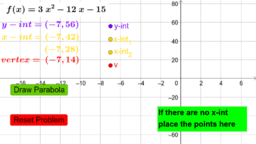### Graphing Quadratic Equations - Practice Exam

Activity

Garret Cahill

•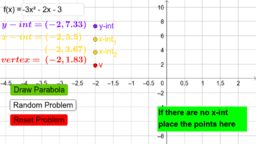Activity

Garret Cahill

•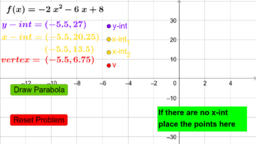### Graphing Quadratic Equations - Homework 4

Activity

Garret Cahill

•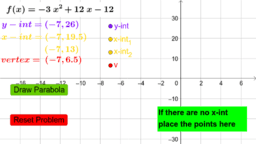### Graphing Quadratic Equations - Homework 3

Activity

Garret Cahill

•### Graphing Quadratic Equations - Classwork Example 4

Activity

Garret Cahill

•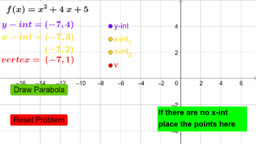### Graphing Quadratic Equations - Classwork Example 3

Activity

Garret Cahill

•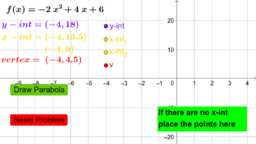### Graphing Quadratic Equations - Classwork Example 2

Activity

Garret Cahill

•### Graphing Quadratic Equations - Classwork Example 1

Activity

Garret Cahill

•### Graphing Quadratic Equations - Homework 4

Activity

Garret Cahill

•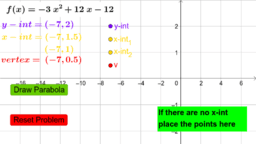### Graphing Quadratic Equations - Homework 3

Activity

Garret Cahill

•### Graphing Quadratic Equations - Homework 2

Activity

Garret Cahill

•Activity

Garret Cahill

•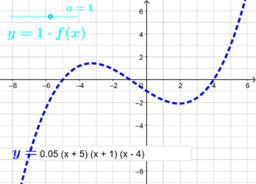### Vertical Dilations with reflections

Activity

Garret Cahill

•### Vertical Dilations with a>0

Activity

Garret Cahill

•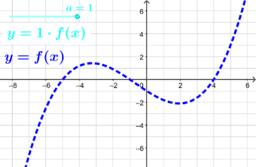### Vertical Dilations with 0<a<1

Activity

Garret Cahill